Financial targets - Fabege

# Financial targetsIn the financial targets of Fabege we are listing; return on equity, loan-to-value ratio, equity/assets ratio, interest coverage ratio and debt ratio.

### Results 2019

Return on equity
Fabege will consistently be among
companies in terms of return on equity.

The return on shareholders’ equity amounted to 16 per cent through contributions from Property
Management and Development.

#### 16%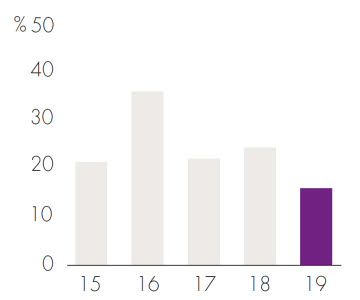Loan-to-value ratio
The loan-to-value ratio must never
exceed 50 per cent.

The loan-to-value ratio fell to 36 per cent due to rising property values and value-creating
investments.

#### 36%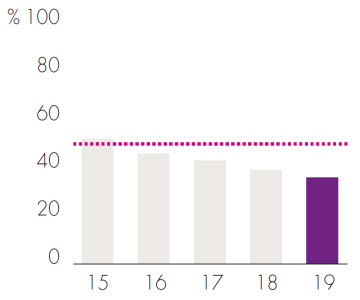Equity/assets ratio
The target is to maintain an equity/assets ratio of at least 35 per cent.

The equity/assets ratio was 52 per cent.

#### 52%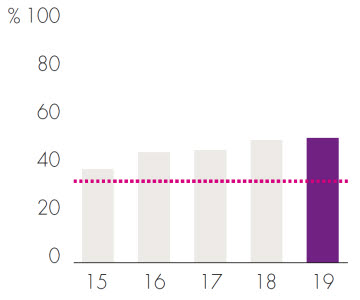Interest coverage ratio
The interest coverage ratio is to be at least 2.2.

The interest coverage ratio is well above the target, an effect of strong net operating income
combined with low market interest rates.

4.4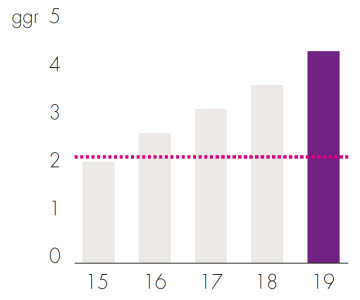Debt ratio
The long term debt ratio will amount to a maximum of 13.

The debt ratio calculated as interest-bearing
liabilities divided by net operating income less central administration amounted to 12.8.

#### 12.8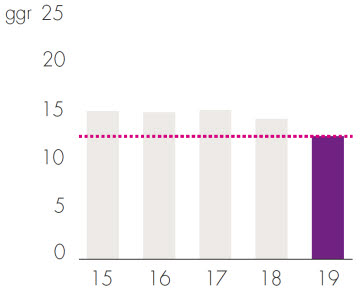Surplus ratio
The target is for the surplus ratio to amount to 75 per cent

The surplus ratio has continually improved, owing
to growing revenue, an increasingly modern portfolio
and persistent efforts to keep costs down.

#### 75%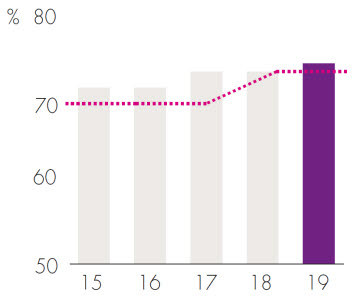Published 17 September 2018